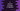# C program to check if a number is a Kaprekar number or not## C program to check if a number is a Kaprekar number or not:

In this C programming tutorial, we will learn how to check if a number is a Kaprekar number or not. The program will take a number as an input from the user and print if it is a Kaprekar number or not.

We will learn what is a Kaprekar number, how to check if a number is a Kaprekar number or not using C.

### Kaprekar number:

To check if a number is a Kaprekar number or not, we have to follow the below steps:

• Find the square of the number.
• Divide the number into two parts.
• If the sum of these two parts is equal to the number itself, it is called a Kaprekar number.

For example, 2223 is a Kaprekar number. The square value of this number is 2223 * 2223 = 4941729. If we divide 4941729 into two parts 494 and 1729, the sum of these two numbers is 2223, i.e. the number itself.

### Algorithm to check if a number is Kaprekar number or not:

We can follow the below algorithm to check if a number is Kaprekar or not:

• Take the number as input from the user.
• Find the square of the number.
• Find the total number of digits in the square value.
• By using a loop, break the square value into two parts and find the sum of these two parts.
• If the sum is equal to the number, it is a Kaprekar number. Else, it is not.

### C program:

Below is the complete C program:

``````#include <stdio.h>

int square(int n)
{
return n * n;
}

int getDigitsCount(int n)
{
int digits = 0;

while (n)
{
digits++;
n /= 10;
}
return digits;
}

int getTenthPow(int pow)
{
int p = 1;
while (pow--)
{
p *= 10;
}
return p;
}

int isKaprekar(int n)
{
int s = square(n);
int digitsCount = getDigitsCount(s);
int sum;

if (n == 1)
{
return 1;
}

for (int i = 1; i < digitsCount; i++)
{
int tenthPow = getTenthPow(i);

if (s % tenthPow == 0)
{
continue;
}

sum = (s / tenthPow) + (s % tenthPow);

if (sum == n)
{
return 1;
}
}
return 0;
}

int main()
{
int no;
printf("Enter a number: ");
scanf("%d", &no);
if (isKaprekar(no))
{
printf("%d is a Kaprekar number\n", no);
}
else
{
printf("%d is not a Kaprekar number\n", no);
}
return 0;
}``````

Here,

• isKaprekar method is used to check if a number is Kaprekar or not.
• It takes a number as the parameter and returns 1 or 0.
• It finds the square of the number and total digits in the square value first.
• If the number is 1, it returns 1 because 1 is a Kaprekar number.
• By using the for loop, it divides the number in two parts and finds the sum of the parts. If the sum is equal to the number itself, it returns 1.
• Else, it returns 0 once the loop completes.

### Sample output:

It will print output as like below:

``````Enter a number: 2728
2728 is a Kaprekar number

Enter a number: 332
332 is not a Kaprekar number

Enter a number: 703
703 is a Kaprekar number``````